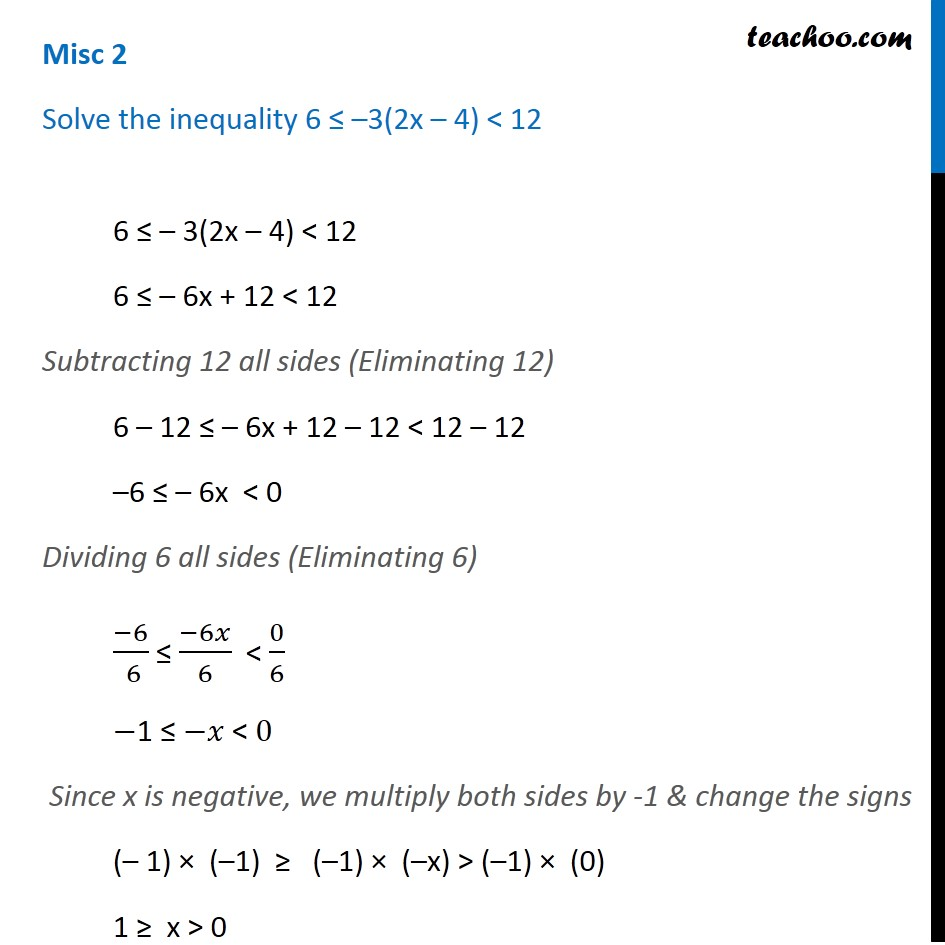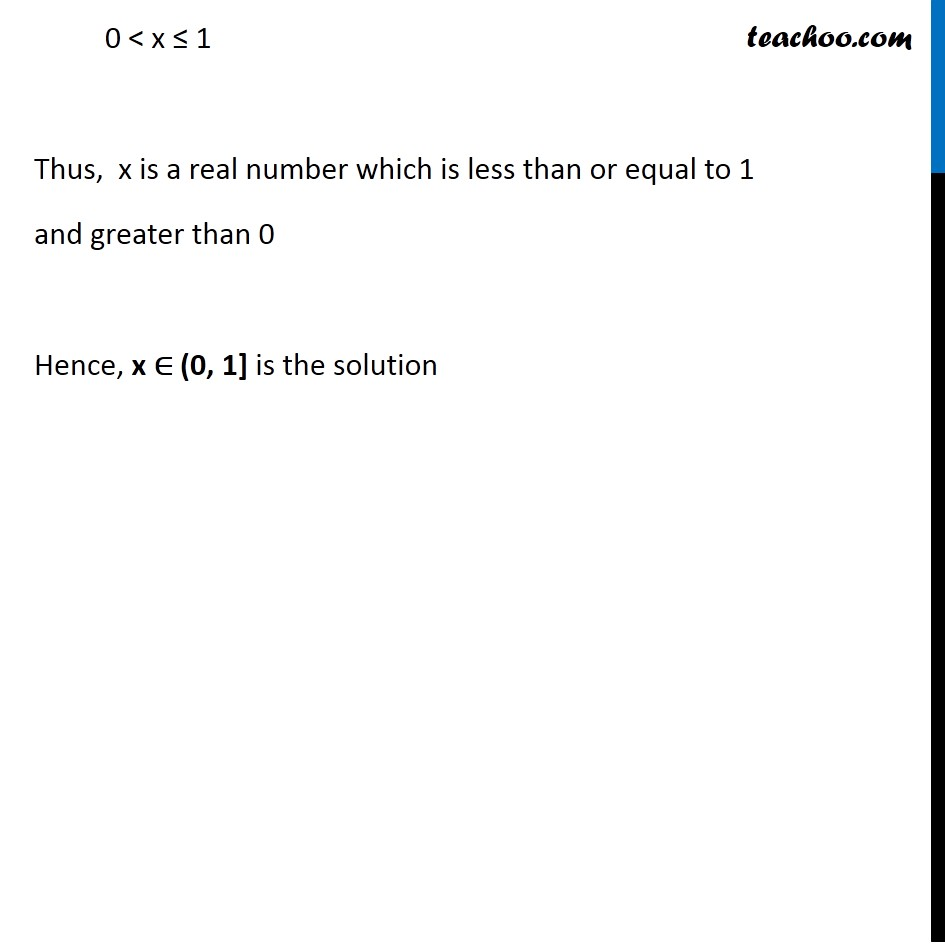Miscellaneous

Chapter 5 Class 11 Linear Inequalities
Serial order wiseLearn in your speed, with individual attention - Teachoo Maths 1-on-1 Class

### Transcript

Misc 2 Solve the inequality 6 ≤ –3(2x – 4) < 12 6 ≤ – 3(2x – 4) < 12 6 ≤ – 6x + 12 < 12 Subtracting 12 all sides (Eliminating 12) 6 – 12 ≤ – 6x + 12 – 12 < 12 – 12 –6 ≤ – 6x < 0 Dividing 6 all sides (Eliminating 6) (−6)/( 6) ≤ (−6𝑥)/6 < 0/6 −1 ≤ −𝑥 < 0 Since x is negative, we multiply both sides by -1 & change the signs (– 1) × (–1) ≥ (–1) × (–x) > (–1) × (0) 1 ≥ x > 0 0 < x ≤ 1 Thus, x is a real number which is less than or equal to 1 and greater than 0 Hence, x ∈ (0, 1] is the solution